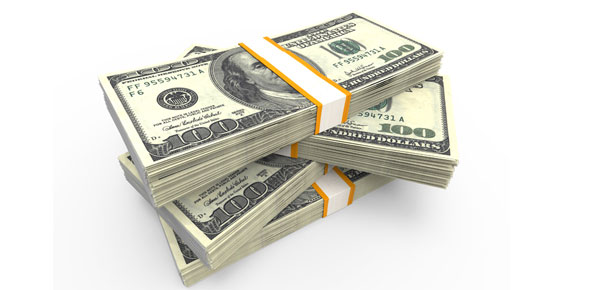# Money Skills Quiz

5 Questions | Total Attempts: 102SettingsThis is quiz to help you better understand the value of money and which type of money is worth more. From coins to dollar bills you will be able to learn the which one is worth more!

Related Topics
• 1.
Which statement will have the correct value of sixty cents (.60)?
• A.

Three dimes

• B.

Five dimes

• C.

Six dimes

• 2.
John has three dollars (\$3.00) and gives one dollar (1.00) to Bill, how many dollars does John have left?
• A.

1

• B.

2

• C.

3

• 3.
A nickle is worth how much?
• A.

5 cents

• B.

10 cents

• C.

25 cents

• 4.
How much is 3 dimes and 2 nickles worth?
• A.

35 cents

• B.

55 cents

• C.

40 cents

• 5.
Which has a great (>) value  4 dimes, 10 nickles, or 2 half dollars?
• A.

4 dimes

• B.

10 nickles

• C.

2 half dollars